﻿ A Theoretical Derivation of Faraday's Second Law of Electromagnetic InductionPublications are Open
Access in this journal
Article Versions
Export Article
• Normal Style
• MLA Style
• APA Style
• Chicago Style
Research Article
Open Access Peer-reviewed

### A Theoretical Derivation of Faraday's Second Law of Electromagnetic Induction

Wei Feng
International Journal of Physics. 2020, 8(4), 120-123. DOI: 10.12691/ijp-8-4-1
Received August 16, 2020; Revised September 19, 2020; Accepted September 28, 2020

### Abstract

Fraday's law of induction is one of the most important laws in electromagnetism. In this paper, based on the curl of electric field vector generated from a moving charge, we show a detailed derivation process, which describes how to derive the rate of change of the magnetic field with time from the curl of the electric field vector. From the mathematical derivation, we found that the acceleration of the charge movement can generate one electric field, which is essentially equivalent to the a non-conservative electric field excited by the ratio of change of magnetic field with time. The theoretical derivation is meaningful to explore the nature of electromagnetic field transformation.

### 1. Introduction

Faraday’s Second Law states that a changing magnetic flux will induce a non-conservative electric field (defined as the vortex electric field) which can vary with time. Faraday’s second law is given below: ε= - △ NФ / △ t, where ε is the electromotive force, Φ is the magnetic flux, N is the number of turns. Many excellent demonstrations and experiments involving Faraday’s law of electromagnetic induction have been discussed during the past several years 1, 2, 3, 4, 5, 6, 7, 8, 9, 10, 11. In many of these experiments, Faraday’s experiment demonstrates that an electric current is induced in the loop by changing the magnetic field. It was also found that the induced voltage (emf) depends on the rate of change of magnetic flux through the coil. However, few papers reported the theoretical analysis of Faraday’s second law. Based on Maxwell’s equation, Faraday’s second law of induction can be easily derived 12. But in fact, the derivation of Maxwell’s equations comes from on Faraday's second law, which cannot be used to prove Faraday's law.

In this article, we describe an approach of mathematical treatment to obtain the rate of change of magnetic field with time by a curl of electric field vector generated by a moving charge with acceleration. Based on this, we naturally derived Faraday’s second law of electromagnetic induction, which will help us better understand the generation mechanism of non-conservative electric field.

### 2. Basic Theoretical Derivation

For the convenience of the following derivation, we discuss the characteristics of the electric field generated by the charge in a two-dimensional plane. For a charge moving at x-direction, the corresponding distribution of electric field is shown in Figure 1.

• Figure 1. Distribution diagram of electric field generated by moving charge in space

For the P-point in Figure 1, the corresponding distribution of electric field vector in the Stationary frame of reference is expressed as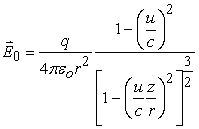(1)

Here E0 is electric field vector, u is the speed of charge q relative to the ground (at x-direction), q is the amount of charge, r is the distance from the origin (O-Point) in the coordinate reference frame to P-point, and z is the projection of r at z axis of stationary frame of reference, ε0 is the dielectric constant in vacuum, c is the speed of light in a vacuum. At P-point in Figure 1, the components of electric field vector at x and z -directions is described as, respectively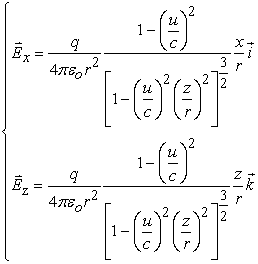(2)

Ex and Ez are the components of electric field vector at x and z-directions in the stationary frame of reference, respectively. Taking the curl of electronic field vector in equation (1) and determinant expansion, we obtain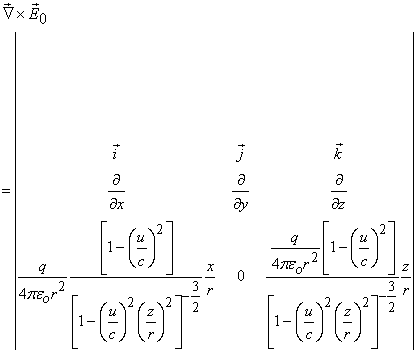(3)

The equation (3) is expanded to be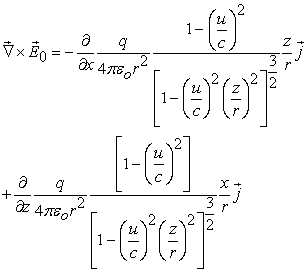(4)

For the charge moving with acceleration at x-direction, the speed u is related to the value of x. So, taking the partial derivatives of equation (4), respectively, we get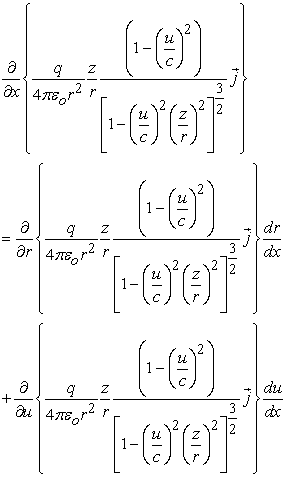(5)

and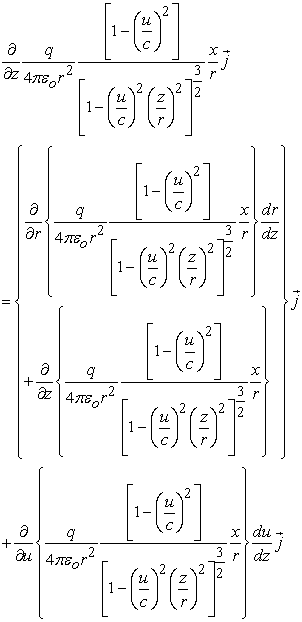(6)

The substitution of equation (5) and (6) into equation yields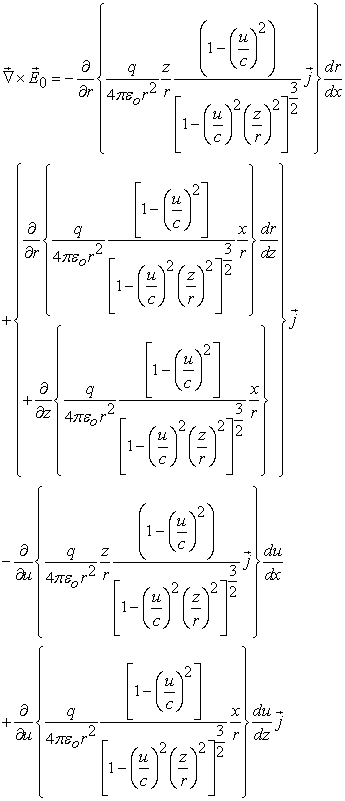(7)

We carefully observe equation (7) and find that the first three terms in the equation actually correspond to the curl of electric field vector generated by a charge q with the instantaneous speed of u, and the latter two terms in equation (7) correspond to the curl of the electric field vector generated by the acceleration of the charge. Here, for the convenience of the following discussion, the first three terms in the equation can be written as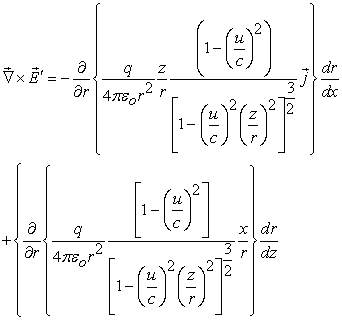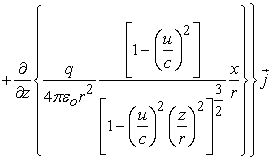(8)

here E’ corresponds to the electric field vector of charges with the speed of u, Substitution of equation (8) into (7) produces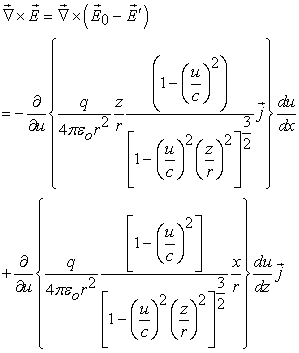(9)

here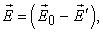obviously, E correspond to the induced electric field vector by the acceleration of the charge.

Using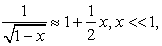the first term in equation (9) can be written approximately as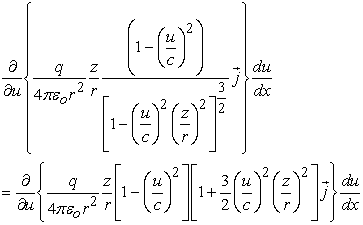(10)

It must be pointed out that the magnetic field is derived by the theory of relativity and ignoring higher-order terms in the theoretical derivation process. Similarly, by ignoring higher-order terms and using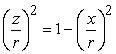in equation (10), the equation can be also written as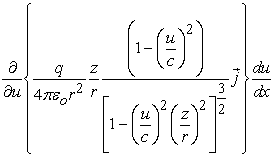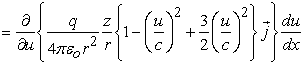(11)

the partial derivative of the equation (11 )generates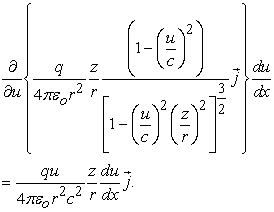(12)

Because the charge only moves at x-direction, the speed u has nothing to do with the value of z, so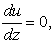thus, the partial derivative of second term in equation (9) is zero, namely,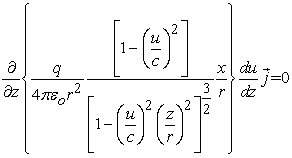(13)

Substitution of equation (12) and (13) into equation (9) produces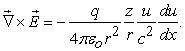(14)

Equation (14) can also be rewritten as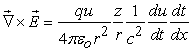(15)

here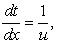u is the speed of charge. Equation (15) is derived under the condition that we all terms except speed are constant. Thus, the equation can be again written as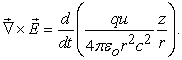(16)

In Figure 1, the corresponding magnetic induction intensity of moving charge in space is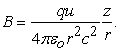(17)

Here B is the magnetic field vector. The substitution of equation (17) into equation (16) yields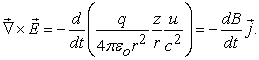(18)

From Mathematical Stoker Formula,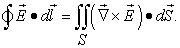(19)

We substitute equation (18) into (19) and get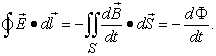(20)

Here Ф is the magnetic flux. Equation (20) is Faraday's law of electromagnetic induction.

From the above derivation process, it is found that the non-conservative electric field vector will be excited by the acceleration of the charge, which is actually equal to that excited by the rate of change of the magnetic field vector with time. That is to say, only an accelerating charge can generate non-conservative electric field vector in space and emit electromagnetic waves into space.

### 3. Conclusion

Using the mathematical expression of curl and Stoke’s equation, We derive the expression of Faraday’s second law, which is completely different from the derivation methods that have been reported. Such a derivation method is meaningful for a deeper understanding of the conversion relationship between electric field and magnetic field.

### References

  Robert Kingman, S.Clark Rowland, Sabin PopeScu, An experimental observation of Faraday’s law of induction, Am. J. Phys. 2002(70) 595-560. In article View Article  A. Singh, Y. N. Mohapatra, and S. Kumar, Electromagnetic induction and damping: Quantitative experiments using a PC interface, Am. J. Phys(2002). 70, 424-427. In article View Article  R. Kingman, S. C. Rowland, and S. Popescu, An experimental observation of Faraday’s law of induction, Am. J. Phys. 2002(70), 595-598. In article View Article  C. C. Jones, Faraday’s law apparatus for the freshmen laboratory, Am. J. Phys. (1987) 55, 1148-1150. In article View Article  J. Barbero, J. A. Manzanares, and S. Mafe, Induced emf in a solenoid: A simple quantitative verification of Faraday’s law, Phys. Educ. (1994)29, 102-105. In article View Article  C. S. MacLatchy, P. Backman, and L. Bogan, A quantitative magnetic breaking experiment, Am. J. Phys. 1993(61), 1096-1101. In article View Article  K. D. Hahn, E. M. Johnson, A. Brokken, and S. Baldwin, Eddy current damping of a magnet moving through a pipe, Am. J. Phys.1998 (66), 1066-1076. In article View Article  R. C. Nicklin, Faraday’s Law and Qualitative Experiments, Am. J. Phys.(1986) 54, 422-428; In article View Article  C. S. MacLatchy, P. Backman, and L. Bogan, A quantitative magnetic breaking experiment, Am. J. Phys.(1993) 61, 1096-1101. In article View Article  J. A. Manzanares, J. Bisquert, G. Garcia-Belmonte, and M. Feranandez Alonso, An experiment on magnetic induction pulses, Am. J. Phys(1994). 62,702-706. In article View Article  P. Carpena, Velocity measurements through magnetic induction, Am. J. Phys (1997). 65, 135-140. In article View Article  Igal Galili, Dov Kaplan, and Yaron Lehavi, Teaching Faraday’s law of electromagnetic induction in an introductory physics course, Am. J. Phys. (2006) 74, 337. In article View ArticleThis work is licensed under a Creative Commons Attribution 4.0 International License. To view a copy of this license, visit http://creativecommons.org/licenses/by/4.0/